Anúncio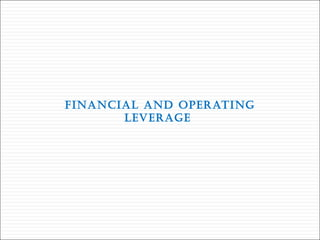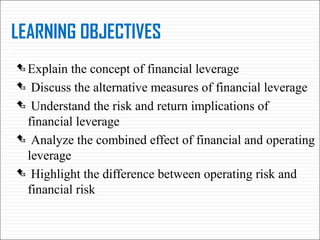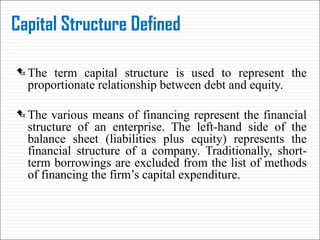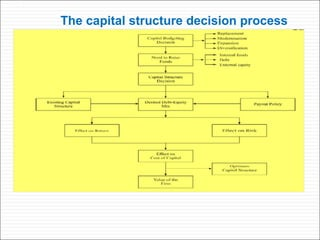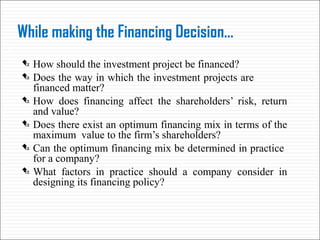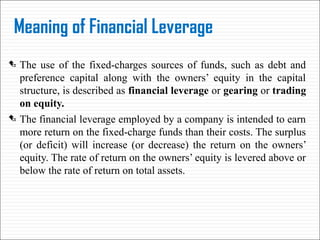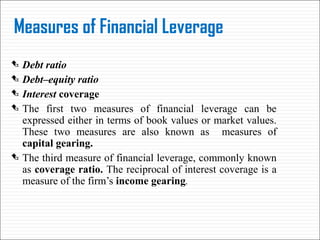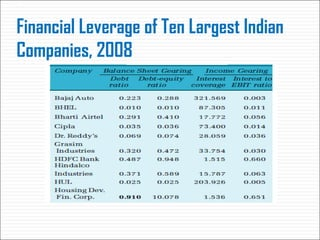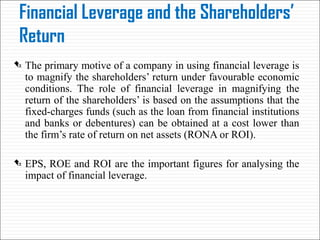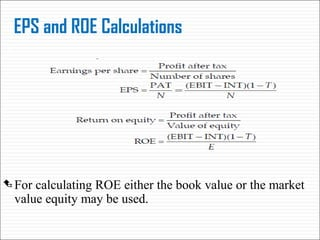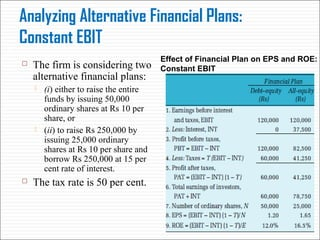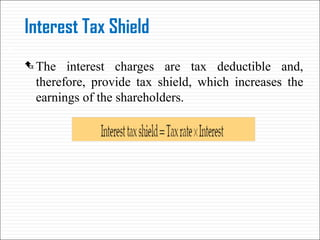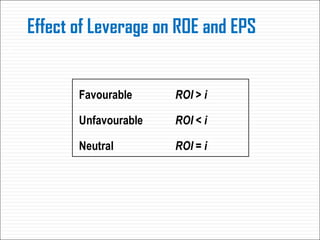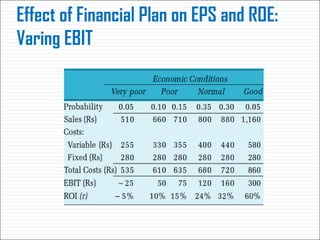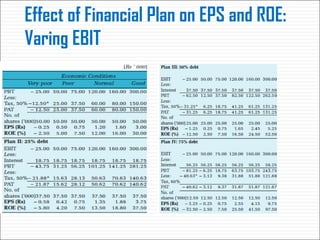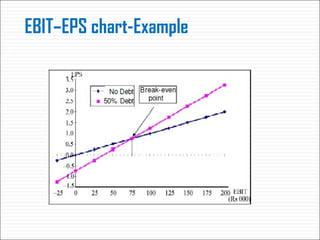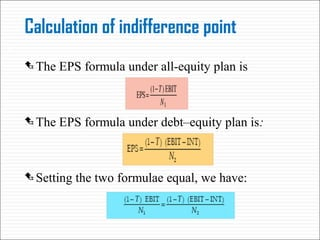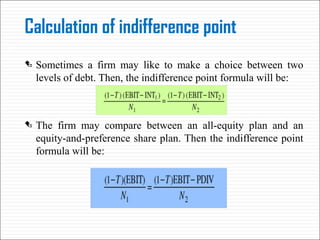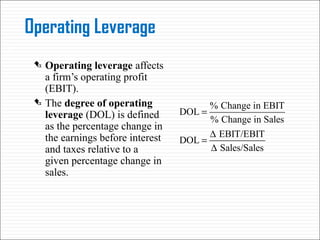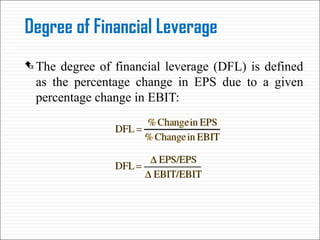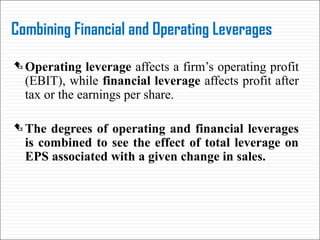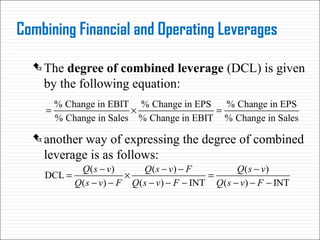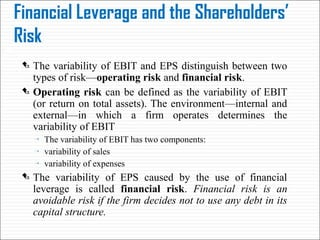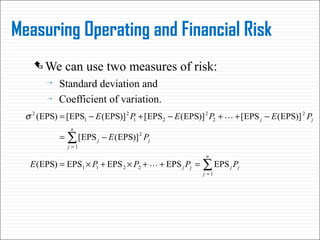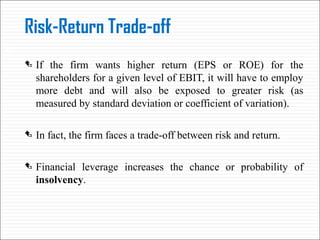1 de 25
Anúncio

### 3 financial leverage

1. FINANCIAL AND OPERATING LEVERAGE
2. LEARNING OBJECTIVES  Explain the concept of financial leverage  Discuss the alternative measures of financial leverage  Understand the risk and return implications of financial leverage  Analyze the combined effect of financial and operating leverage  Highlight the difference between operating risk and financial risk 2
3. Capital Structure Defined  The term capital structure is used to represent the proportionate relationship between debt and equity.  The various means of financing represent the financial structure of an enterprise. The left-hand side of the balance sheet (liabilities plus equity) represents the financial structure of a company. Traditionally, short- term borrowings are excluded from the list of methods of financing the firm’s capital expenditure. 3
4. 4 The capital structure decision process
5. While making the Financing Decision...  How should the investment project be financed?  Does the way in which the investment projects are financed matter?  How does financing affect the shareholders’ risk, return and value?  Does there exist an optimum financing mix in terms of the maximum value to the firm’s shareholders?  Can the optimum financing mix be determined in practice for a company?  What factors in practice should a company consider in designing its financing policy? 5
6. Meaning of Financial Leverage  The use of the fixed-charges sources of funds, such as debt and preference capital along with the owners’ equity in the capital structure, is described as financial leverage or gearing or trading on equity.  The financial leverage employed by a company is intended to earn more return on the fixed-charge funds than their costs. The surplus (or deficit) will increase (or decrease) the return on the owners’ equity. The rate of return on the owners’ equity is levered above or below the rate of return on total assets. 6
7. Measures of Financial Leverage  Debt ratio  Debt–equity ratio  Interest coverage  The first two measures of financial leverage can be expressed either in terms of book values or market values. These two measures are also known as measures of capital gearing.  The third measure of financial leverage, commonly known as coverage ratio. The reciprocal of interest coverage is a measure of the firm’s income gearing. 7
8. 8 Financial Leverage of Ten Largest Indian Companies, 2008
9. Financial Leverage and the Shareholders’ Return  The primary motive of a company in using financial leverage is to magnify the shareholders’ return under favourable economic conditions. The role of financial leverage in magnifying the return of the shareholders’ is based on the assumptions that the fixed-charges funds (such as the loan from financial institutions and banks or debentures) can be obtained at a cost lower than the firm’s rate of return on net assets (RONA or ROI).  EPS, ROE and ROI are the important figures for analysing the impact of financial leverage. 9
10. EPS and ROE Calculations  For calculating ROE either the book value or the market value equity may be used. 10
11. Analyzing Alternative Financial Plans: Constant EBIT  The firm is considering two alternative financial plans:  (i) either to raise the entire funds by issuing 50,000 ordinary shares at Rs 10 per share, or  (ii) to raise Rs 250,000 by issuing 25,000 ordinary shares at Rs 10 per share and borrow Rs 250,000 at 15 per cent rate of interest.  The tax rate is 50 per cent. 11 Effect of Financial Plan on EPS and ROE: Constant EBIT
12. Interest Tax Shield  The interest charges are tax deductible and, therefore, provide tax shield, which increases the earnings of the shareholders. 12
13. Effect of Leverage on ROE and EPS Favourable ROI > i Unfavourable ROI < i Neutral ROI = i 13
14. Effect of Financial Plan on EPS and ROE: Varing EBIT14
15. Effect of Financial Plan on EPS and ROE: Varing EBIT15
16. EBIT–EPS chart-Example 16
17. Calculation of indifference point  The EPS formula under all-equity plan is  The EPS formula under debt–equity plan is:  Setting the two formulae equal, we have: 17
18.  Sometimes a firm may like to make a choice between two levels of debt. Then, the indifference point formula will be:  The firm may compare between an all-equity plan and an equity-and-preference share plan. Then the indifference point formula will be: 18 Calculation of indifference point
19. Operating Leverage  Operating leverage affects a firm’s operating profit (EBIT).  The degree of operating leverage (DOL) is defined as the percentage change in the earnings before interest and taxes relative to a given percentage change in sales. 19 % Change in EBIT DOL % Change in Sales EBIT/EBIT DOL Sales/Sales = ∆ = ∆
20. Degree of Financial Leverage  The degree of financial leverage (DFL) is defined as the percentage change in EPS due to a given percentage change in EBIT: 20
21. Combining Financial and Operating Leverages  Operating leverage affects a firm’s operating profit (EBIT), while financial leverage affects profit after tax or the earnings per share.  The degrees of operating and financial leverages is combined to see the effect of total leverage on EPS associated with a given change in sales. 21
22. Combining Financial and Operating Leverages  The degree of combined leverage (DCL) is given by the following equation:  another way of expressing the degree of combined leverage is as follows: 22 % Change in EBIT % Change in EPS % Change in EPS % Change in Sales % Change in EBIT % Change in Sales = × = ( ) ( ) ( ) DCL ( ) ( ) INT ( ) INT Q s v Q s v F Q s v Q s v F Q s v F Q s v F − − − − = × = − − − − − − − −
23. Financial Leverage and the Shareholders’ Risk  The variability of EBIT and EPS distinguish between two types of risk—operating risk and financial risk.  Operating risk can be defined as the variability of EBIT (or return on total assets). The environment—internal and external—in which a firm operates determines the variability of EBIT  The variability of EBIT has two components:  variability of sales  variability of expenses  The variability of EPS caused by the use of financial leverage is called financial risk. Financial risk is an avoidable risk if the firm decides not to use any debt in its capital structure. 23
24. Measuring Operating and Financial Risk  We can use two measures of risk:  Standard deviation and  Coefficient of variation. 24 2 2 2 2 1 1 2 2 2 1 1 1 2 2 1 (EPS) [EPS (EPS)] [EPS (EPS)] [EPS (EPS)] [EPS (EPS)] (EPS) EPS EPS EPS EPS j j n j j j n j j j j j E P E P E P E P E P P P P σ = = = − + − + + − = − = × + × + + = ∑ ∑ L L
25. Risk-Return Trade-off  If the firm wants higher return (EPS or ROE) for the shareholders for a given level of EBIT, it will have to employ more debt and will also be exposed to greater risk (as measured by standard deviation or coefficient of variation).  In fact, the firm faces a trade-off between risk and return.  Financial leverage increases the chance or probability of insolvency. 25
Anúncio